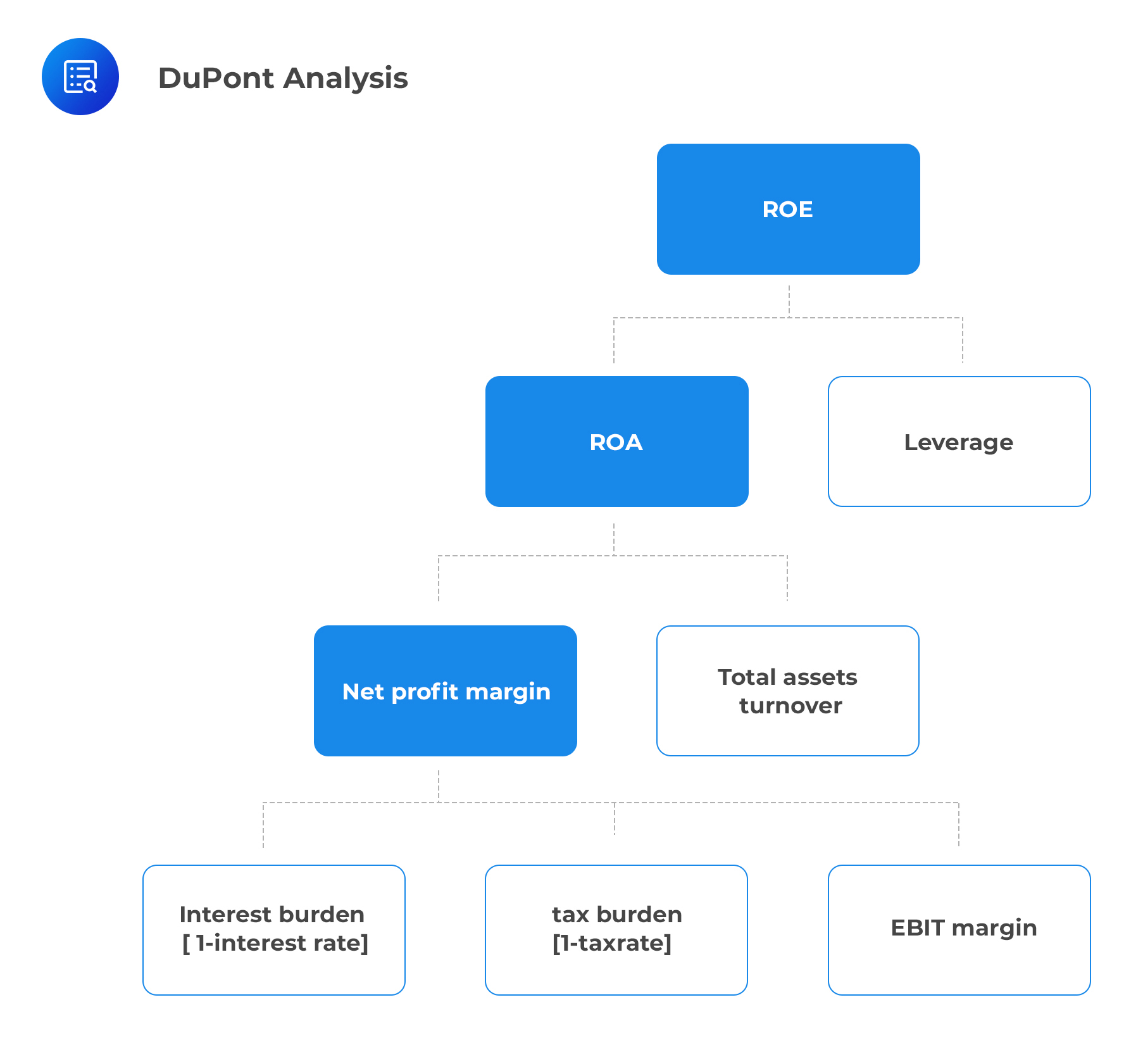# DuPont Analysis of Return on Equity

Return on Equity (ROE), i.e., net income divided by average shareholders’ equity, measures the return that a company generates on its equity capital.

DuPont analysis is a technique that can be used to decompose ROE into its constituent parts. The process involves the expression of the basic ratio as the product of component ratios. This decomposition is useful in the determination of the reasons for changes in ROE over time for a given company. It also demystifies the differences in ROE for different companies in a given time period.

The figure below illustrates the idea behind DuPont’s analysis.## Application of Dupont Analysis

There are several methods for decomposing ROE using simple algebra.

### Method 1:

\begin{align}\text{ROE} &= \frac{\text{Net income}}{ \text{Average shareholders’ equity}}\\ &= \left(\frac{\text{Net income}} {\text{Average total assets}}\right)\times \left(\frac{\text{Average total assets}}{\text{Average shareholders’ equity}}\right)\end{align}

Therefore

$$\text{ROE} = \text{Return on Assets (ROA)} \times \text{Leverage}$$

This implies that when a company uses leverage, a higher ROA will lead to a higher ROE.

### Method 2:

\begin{align}\text{ROE} &= \frac{\text{Net income}}{\text{Average shareholders’ equity}}\\ &= \left(\frac{\text{Net income}}{ \text{Revenue}}\right) \times \left(\frac{\text{Revenue} }{\text{Average total assets}}\right)\times \left(\frac{\text{Average total assets}}{\text{Average shareholders’ equity}}\right)\end{align}

Which is interpreted as:

$$\text{ROE} = \text{Net profit margin} \times \text{Total asset turnover}\times \text{Leverage}$$

This tells us that when a company uses leverage, a higher asset turnover and net profit margin will lead to a higher return on equity.

### Method 3:

To further separate the effects of taxes and interest, the net profit margin can further be decomposed leading to:

\begin{align}\text{ROE} = &\frac{\text{Net income}}{\text{Average shareholders’ equity}}\\= &\left(\frac{\text{Net income}}{ \text{EBT}}\right) \times \left(\frac{\text{EBT}}{\text{EBIT}}\right) \times \left(\frac{\text{EBIT}}{ \text{Revenue}}\right) \times\\ &\left(\frac{\text{Revenue}}{\text{Average total assets}}\right) \times \left(\frac{\text{Average total assets}}{\text{Average shareholders’ equity}}\right)\\ & = \text{Tax burden}\times \text{Interest burden} \times \text{EBIT margin}\times \text{Total asset turnover} \times \text{Leverage} \end{align}

This 5-way decomposition is the Dupont Analysis method that is found in financial databases such as Bloomberg.

### Question 1

Given the following financial data on a company, what is the company’s ROE?

$$\begin{array} {ll}\\ \text{Net income}&50,000\\ \text{Revenue}&2,85,000\\ \text{Average total assets}&1,000,000\\ \text{Average shareholder’s equity}& 600,000\\ \end{array}$$

1. 5.29%
2. 8.33%
3. 1.14%

Solution

\begin{align}\text{ROE} &= \left(\frac{\text{Net income}}{\text{Revenue}}\right) × \left(\frac{\text{Revenue}}{\text{Average total assets}}\right) × \left(\frac{\text{Average total assets}}{\text{Average shareholders’ equity}}\right)\\&= \frac{50,000}{285,000} × \frac{285,000}{1,000,000} × \frac{1,000,000}{600,000} = 0.1754 × 0.285 × 1.667 = 8.33\%.\end{align}

Shop CFA® Exam Prep

Offered by AnalystPrepLevel I
Level II
Level III
All Three Levels
Featured Shop FRM® Exam PrepFRM Part I
FRM Part II
FRM Part I & Part II
Learn with Us

Subscribe to our newsletter and keep up with the latest and greatest tips for success
Shop Actuarial Exams PrepExam P (Probability)
Exam FM (Financial Mathematics)
Exams P & FM
Shop GMAT® Exam PrepComplete CourseSergio Torrico
2021-07-23
Excelente para el FRM 2 Escribo esta revisión en español para los hispanohablantes, soy de Bolivia, y utilicé AnalystPrep para dudas y consultas sobre mi preparación para el FRM nivel 2 (lo tomé una sola vez y aprobé muy bien), siempre tuve un soporte claro, directo y rápido, el material sale rápido cuando hay cambios en el temario de GARP, y los ejercicios y exámenes son muy útiles para practicar.diana
2021-07-17
So helpful. I have been using the videos to prepare for the CFA Level II exam. The videos signpost the reading contents, explain the concepts and provide additional context for specific concepts. The fun light-hearted analogies are also a welcome break to some very dry content. I usually watch the videos before going into more in-depth reading and they are a good way to avoid being overwhelmed by the sheer volume of content when you look at the readings.Kriti Dhawan
2021-07-16
A great curriculum provider. James sir explains the concept so well that rather than memorising it, you tend to intuitively understand and absorb them. Thank you ! Grateful I saw this at the right time for my CFA prep.nikhil kumar
2021-06-28
Very well explained and gives a great insight about topics in a very short time. Glad to have found Professor Forjan's lectures.Marwan
2021-06-22
Great support throughout the course by the team, did not feel neglectedBenjamin anonymous
2021-05-10
I loved using AnalystPrep for FRM. QBank is huge, videos are great. Would recommend to a friendDaniel Glyn
2021-03-24
I have finished my FRM1 thanks to AnalystPrep. And now using AnalystPrep for my FRM2 preparation. Professor Forjan is brilliant. He gives such good explanations and analogies. And more than anything makes learning fun. A big thank you to Analystprep and Professor Forjan. 5 stars all the way!michael walshe
2021-03-18
Professor James' videos are excellent for understanding the underlying theories behind financial engineering / financial analysis. The AnalystPrep videos were better than any of the others that I searched through on YouTube for providing a clear explanation of some concepts, such as Portfolio theory, CAPM, and Arbitrage Pricing theory. Watching these cleared up many of the unclarities I had in my head. Highly recommended.